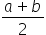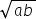Maths-
General
Easy

###Hint:

We know that A.M is Arithmetic Mean and G.M is Geometric Mean and the A.M and G.M for two positive numbers, say a and b will beandrespectively. We will make a quadratic equation using it and the roots of the equation gives the value of the numbers which we have to prove.

#### Detailed SolutionAM between two positive numbers is given byGM between two positive numbers a and b is given byWe have been given that :We have to be careful while solving quadratic equation questions as there are chances of mistakes with the signs while finding the roots. Sometimes the students try to use factorisation methods to solve the quadratic equation, but in this type of questions, it is not recommended at all. Always try to use the quadratic formula for solving. You can also remember the statement to be proved, given in the question as a property for two positive numbers.

## Book A Free Demo+91